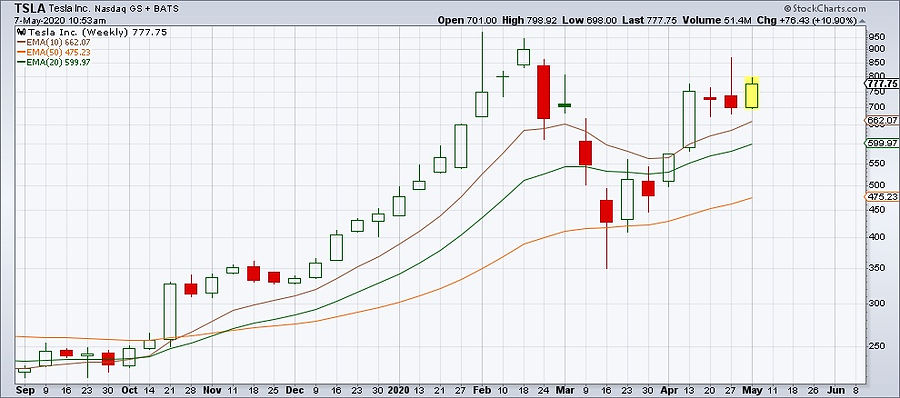top of page

# Moving Averages

A moving average is simply the average price of a security over a certain time period. So, we can calculate the ten-day moving average of a security by adding the price of that stock over the last ten days and divide it by ten.

The first, and probably the most important, use of moving averages comes directly from its construction. Before I explain what I mean, let us remind ourselves that everybody knows the price of most securities contains a lot of “noise”.

By that I mean that price fluctuates round a general trend, if there is one. As a moving average takes the average of prices, it smoothes out any price variations and enables us to see whether the price has any trend.

Therefore, moving averages can only tell us what prices have done on average over the set time period, but not what they will do in the future.

In general moving averages can be useful to:

1. Identify a trend

2. Establish Support & Resistance levels

3. Determine price momentum

4. Formulate a stop-loss or a trailing stop

5. Smooth out volume and indicators

We will explain now in more details these five applications of the moving averages.

1. Identify a trend

Moving averages can be used to identify an uptrend. When the trend is bullish the following are happening:

1. The moving averages are rising

2. Prices generally move above the moving averages

3. The faster moving average is moving above the slower moving average

The opposite is true for a downtrend:

1. The moving averages are falling

2. Prices generally move below the moving averages

3. The faster moving average is moving below the slower moving average

Let’s look at an example:

Fig 1: Moving averages identify the trend

In the chart above we show a region in the green box where there is a bullish trend. There, the three conditions described above are true. More particularly:

1.  The 50- day exponential moving average, the black line, is rising

2. Prices stay above the 50- day exponential moving average.

3. A shorter 20- day exponential moving average, the red line is moving above the longer 50- day exponential moving average throughout the uptrend.

When the trend reversed, the brown box, the faster 20-day moving average moved below the slower 50-day moving average and price moved below both moving averages.

2. Establish Support & Resistance levels

Traders have found that popular moving averages often act as curved or dynamic support or resistance level. When a security approaches a moving average experienced traders wait for reaction signs on behalf of the market participants.

More particularly, traders want to see whether the price will penetrate through the moving average or bounce off and reverse. Both of these outcomes represent trading opportunities.

Here’s an example:

Fig 2:  A moving average acting as support line in an uptrend and as resistance line in a downtrend

We can see in the above chart the points - in the blue circles - where price touched the 20-day moving average and bounced up in the uptrend. Here the moving average acted as a support line. In the same chart, price touched the falling moving average - in the green circles - and bounced down. In this case, the moving average acted as a resistance line.

As support or resistance lines, moving averages have certain advantages over the conventional trendlines we draw for support and resistance. First of all, they are more objective as we don’t draw them ourselves. Second, they are more flexible and follow price fluctuations.

3. Determine price momentum

When momentum starts to build up in either direction, we normally observe the following:

1. Price crosses above (or below) the faster moving average

2. The faster-moving average crosses above (or below) the slower moving average

3. The two moving averages slop up (or down) and diverge from each other as momentum builds up

The above conditions can help us determine the momentum direction as well as its strength.

Fig 3:  In the chart above, price moves above its 10-day exponential moving average. What is more, the 10- 20- and 50-day exponential moving averages are all sloping up and diverging from each other, which are signs of strong bullish momentum.

4. Formulate a stop-loss or a trailing stop

Fig 4:  In this case, we have used the 50-period exponential moving average in the 4-hour chart as a trailing stop.

A suitable moving average below a bullish trend is a usual form of trailing stop. We sell when the price falls below the moving average. An alternative is to wait for the price to close below the moving average for two or three consecutive days.

5. Smooth out volume and indicators

Another application of moving averages is to smooth out an indicator. First of all, the “signal line” of MACD is by default its 9-day moving average!

We also make use of moving averages in Relative Strength, for the same reasons we use moving averages in general. We want to see the Relative Strength line move up and above its 20-day moving average.

Finally, we use the 20- day moving average of Volume to be able to see when our volume is above or below average and when it’s going up or down.

Fig 5:  In the above chart we use the 20-day exponential moving average of Relative Strength and MFI to smooth out the fluctuations and to get a better picture of their movement. In addition to this, the Signal Ling of MACD is the 9-period moving average of MACD.bottom of page